Function Repository Resource:

# ImageSquareDivide

Divide an image into an array of colored squares

Contributed by: Erik Mahieu
 ResourceFunction["ImageSquareDivide"][img] divides the image img into colored squares. ResourceFunction["ImageSquareDivide"][img,reg] divides the image img into squares, retaining only the squares that are members of the region reg.

## Details

If the image is m pixels wide, then the result is a list of m2 squares.
This function is very important for transformations of an image that operate differently on each part of the image. This is the case with anamorphic transformations where the deformation of the original is dependent on the location of each pixel.

## Examples

### Basic Examples (2)

Divide a 2×2 image into squares:

 In:=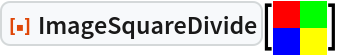Out=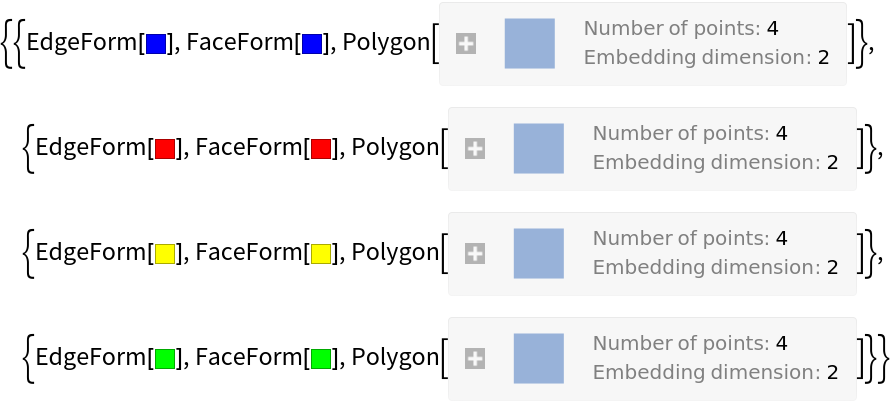Divide an image that is 30 pixels wide into an array of 900 colored square polygons:

 In:=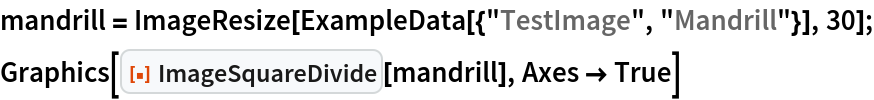Out=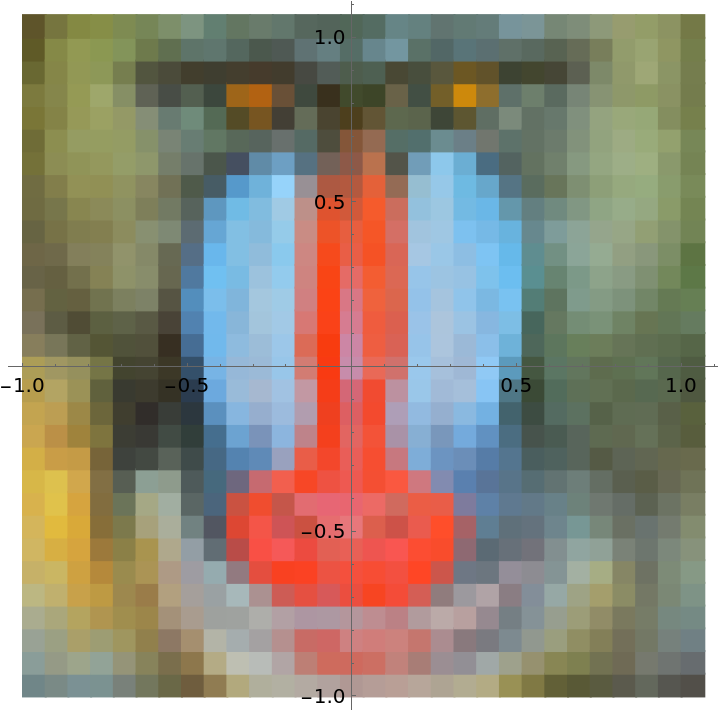Include only those squares that are members of a triangular region:

 In:=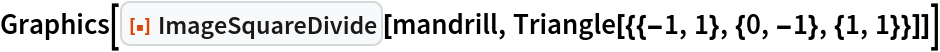Out=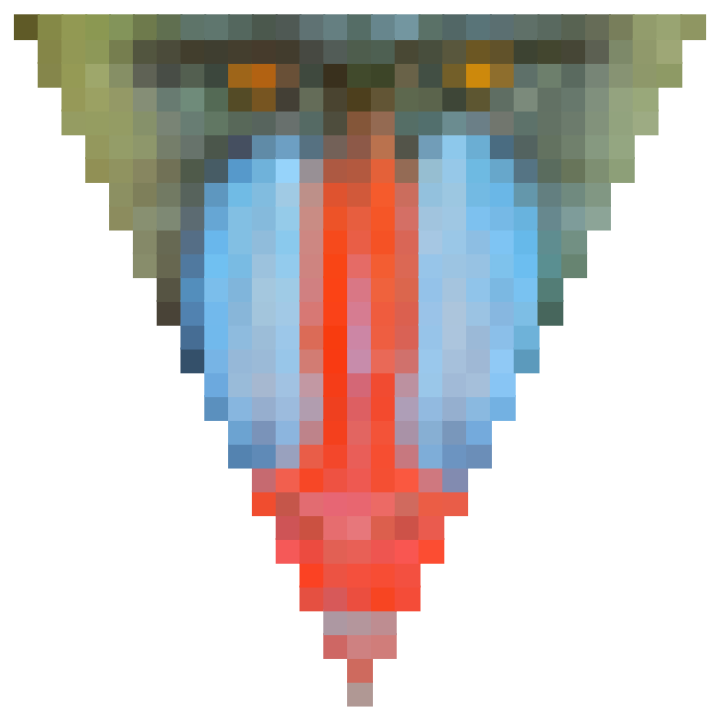### Scope (2)

Different regions can be specified:

 In:=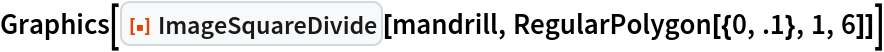Out=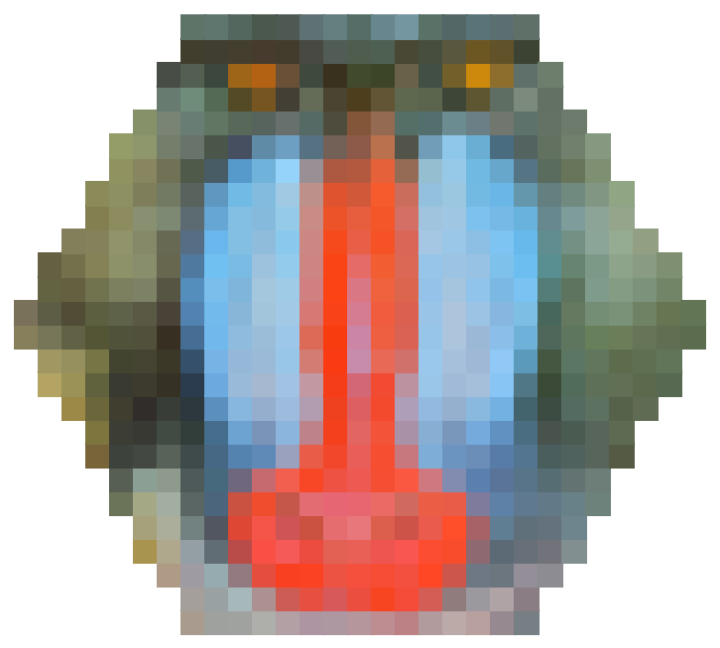The output can be combined with other graphics primitives:

 In:=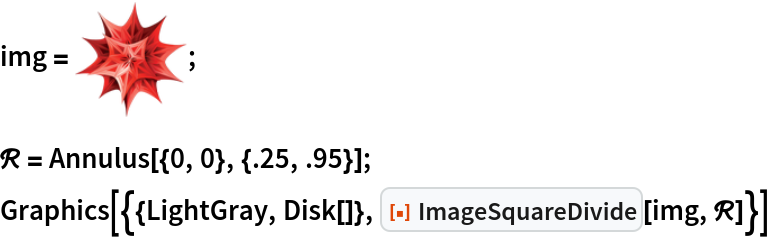Out=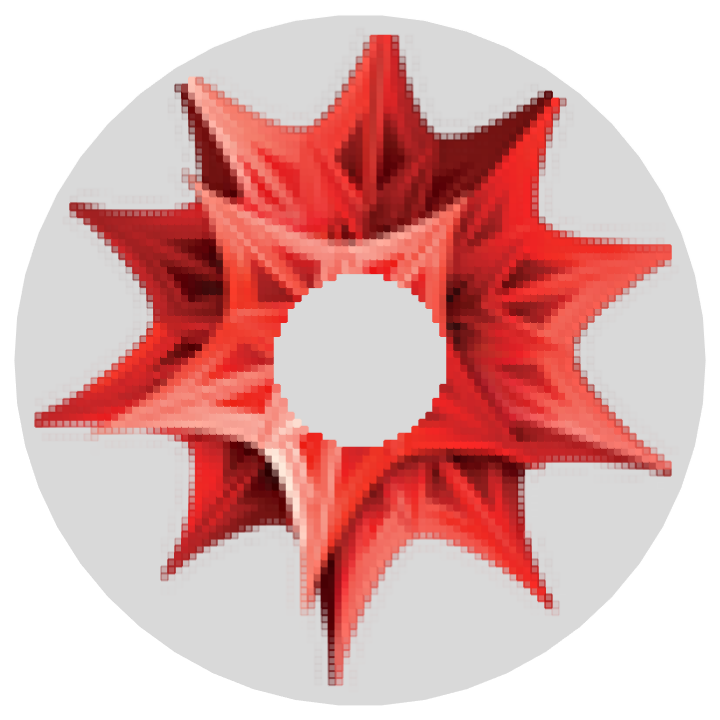### Properties and Relations (1)

The output of the function for an image of width w is a list of w2 colored squares:

 In:=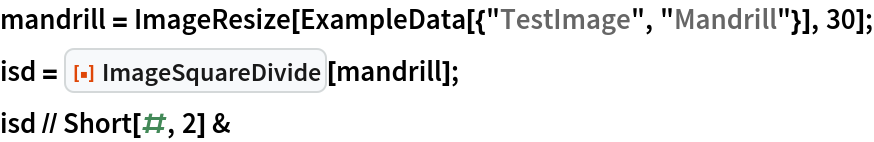Out=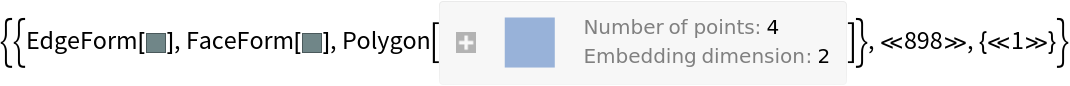In:=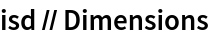Out=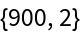### Possible Issues (1)

To be useful, regions should be sized to fit within the Rectangle[{-1,-1},{1,1}] to include the full image:

 In:=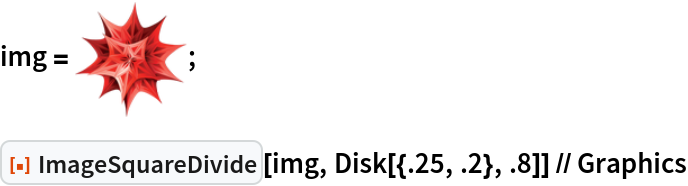Out=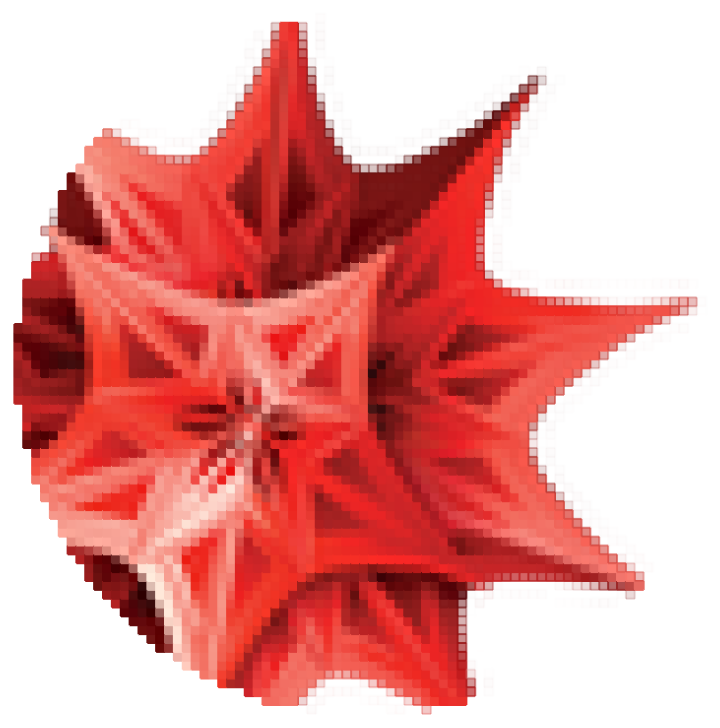### Neat Examples (1)

Here is an example of a conical mirror anamorphic function operating on the vertices of an image that was divided into squares:

 In:=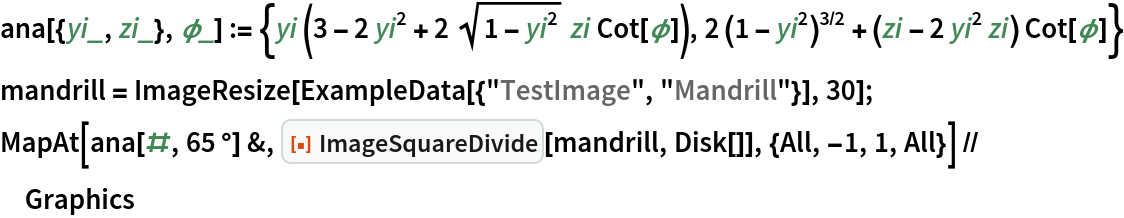Out=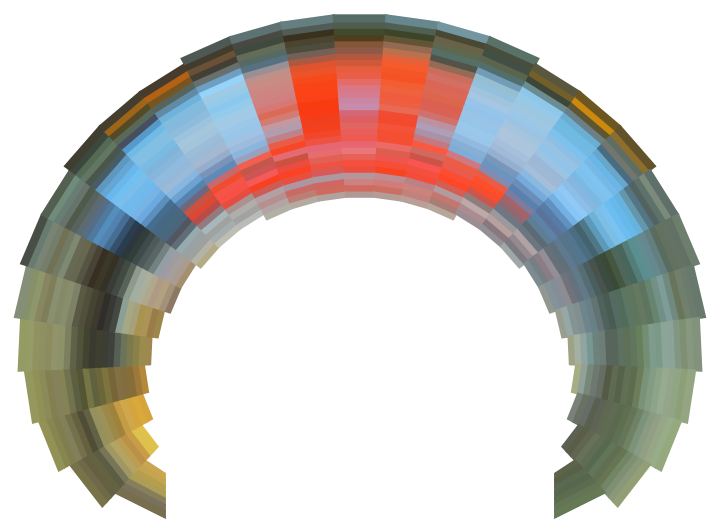Erik Mahieu

## Version History

• 2.0.0 – 01 March 2021
• 1.0.0 – 01 December 2020